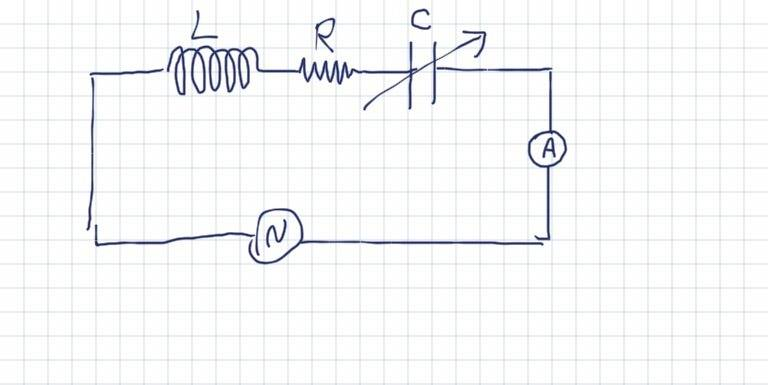# The current in RLC circuit.

## Homework Statement

In the tuned circuit below, what will happen to the reading of the ammeter when:
a) source frequency increases. The voltage is constant
b) the capacitance increases. The voltage and source frequency are constant
c) The voltage increases. Source frequency is constant.## Homework Equations

ƒ =1/(2π√LC) Where: C is the capacitance and L is the inductance.
I= V/Z Where: V is the voltage and Z is the impedance.
Xc=1/ωC
Xl=ωL
Z=√R²+(Xl-Xc)²

## The Attempt at a Solution

Here is my solution:

a) the ammeter reading decreses.
Explanation: when source frequency varies the circuit is no longer in resonance so the impedance increases,
Z=√R²+ (Xl-Xc)²

b) the ammeter reading decreases.
Explanation: when the capacitance C increases, Xc decrease, then (Xl-Xc) increses, hence the impedance increases because:
Z= √R²+(Xl-Xc)²

c) the ammeter reading increases.
Explanation: since source frequency is constant, the circuit is still in resonance. So, increasing in voltage will increase the current becuase the impedance of the circuit will not change.

Am I correct?

Homework Helper

## Homework Statement

In the tuned circuit below, what will happen to the reading of the ammeter when:
a) source frequency increases. The voltage is constant
b) the capacitance increases. The voltage and source frequency are constant
c) The voltage increases. Source frequency is constant.## Homework Equations

ƒ =1/(2π√LC) Where: C is the capacitance and L is the inductance.
I= V/Z Where: V is the voltage and Z is the impedance.
Xc=1/ωC
Xl=ωL
Z=√R²+(Xl-Xc)²

## The Attempt at a Solution

Here is my solution:

a) the ammeter reading decreses.
Explanation: when source frequency varies the circuit is no longer in resonance so the impedance increases,
Z=√R²+ (Xl-Xc)²

b) the ammeter reading decreases.
Explanation: when the capacitance C increases, Xc decrease, then (Xl-Xc) increses, hence the impedance increases because:
Z= √R²+(Xl-Xc)²

c) the ammeter reading increases.
Explanation: since source frequency is constant, the circuit is still in resonance. So, increasing in voltage will increase the current becuase the impedance of the circuit will not change.

Am I correct?
Yes, it is correct. Well done!